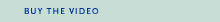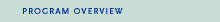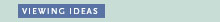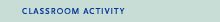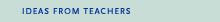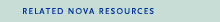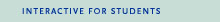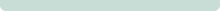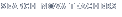Back to Teachers HomeProof, TheViewing IdeasBefore Watching

1. Let students explore the equation xn + yn = zn by substituting different values for n. Have them begin with n = 2, resulting in the familiar Pythagorean Theorem, x2 + y2 = z2. Then have students change the value of n to numbers greater than 2 to see whether they can find solutions that will result in a balanced equation with a positive integer answer for z.

2. To be sure that xn + yn = zn has no solutions when n is a positive integer greater than 2, every value for n needs to be checked—a nearly impossible feat, even with the aid of a computer. A proof is needed. Review with students the concept of theorem, a mathematical statement that can be proved, or awaits proving. Then ask them to describe ways that things can be proved in non-mathematical contexts, such as in law, mystery novels, or in their everyday lives. How do these types of proofs compare to mathematical proofs?

After Watching

1. Ask students to list the traits Anderw Wiles needed to solve Fermat's Last Theorem. Which traits seem most important? Which seem least important?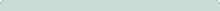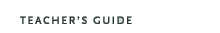Proof, The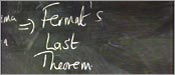Original broadcast:October 28, 1997